maths > trigonometry

Triangular Form of Trigonometric Ratios

what you'll learn...

Overview

»  similar right-triangle

→  given angle $\theta$$\theta$

→  position of right-angle with reference to given angle

→  hypotenuse with reference to the right-angle

→  position of a side with reference to the given angle : opposite-side

→  position of another side with reference to the given angle : adjacent-side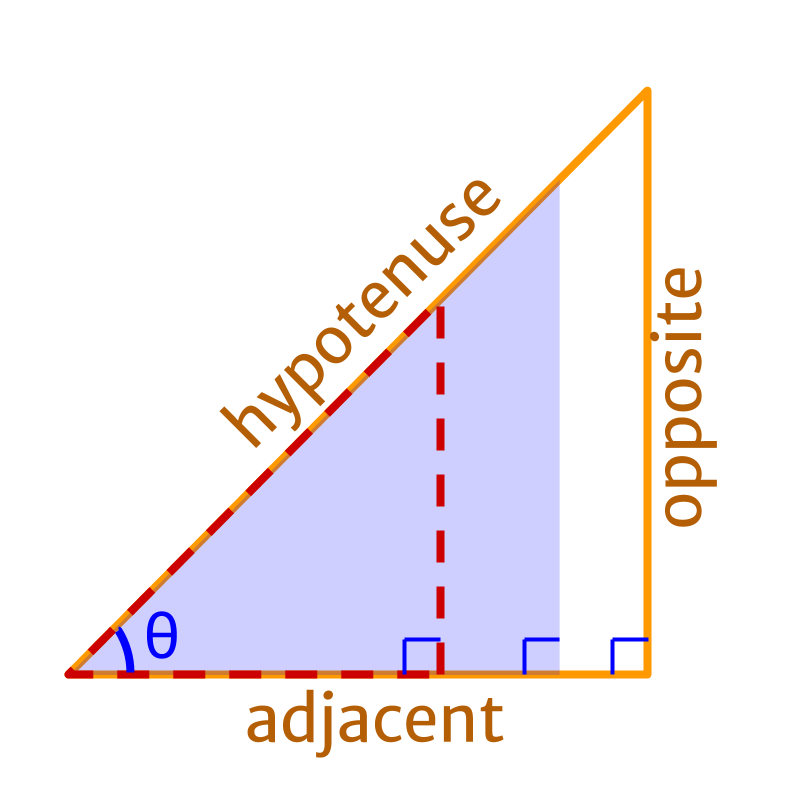»  Trigonometric Ratios
→  $\mathrm{sin}\theta =\frac{\text{opposite}}{\text{hypotenuse}}$$\sin \theta = \frac{\textrm{o p p o s i t e}}{\textrm{h y p o t e \nu s e}}$
→  $\mathrm{cos}\theta =\frac{\text{adjacent}}{\text{hypotenuse}}$$\cos \theta = \frac{\textrm{a \mathrm{dj} a c e n t}}{\textrm{h y p o t e \nu s e}}$
→  $\mathrm{tan}\theta =\frac{\text{opposite}}{\text{adjacent}}$$\tan \theta = \frac{\textrm{o p p o s i t e}}{\textrm{a \mathrm{dj} a c e n t}}$ $\quad = \frac{\sin \theta}{\cos \theta}$

Note: In advanced trigonometry, a general form "trigonometric values on unit circle" will be introduced.

Let us quickly review the terminology in trigonometric ratios.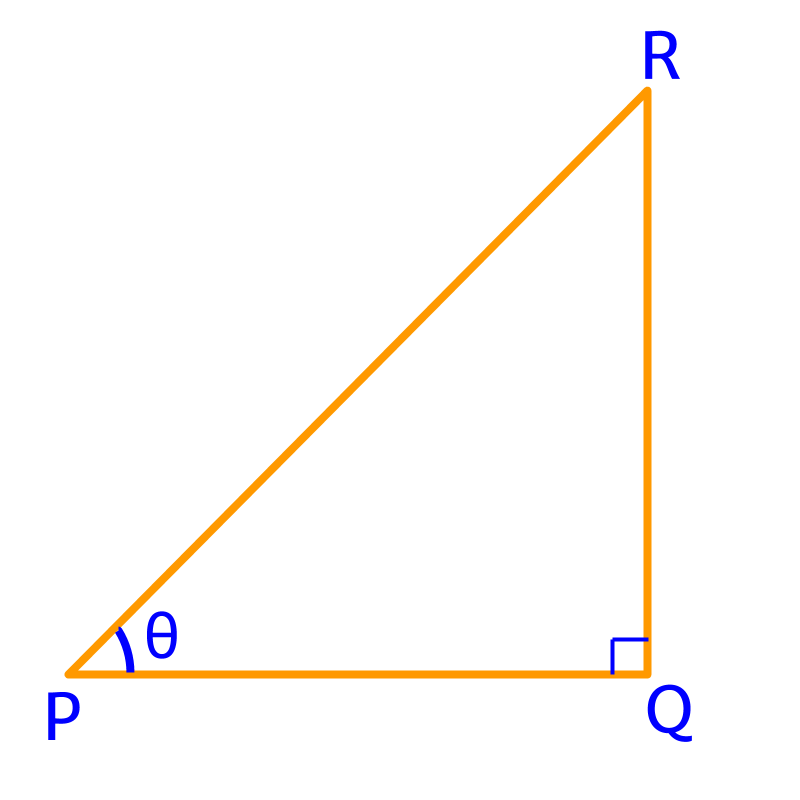In the given figure, $\overline{PR}$$\overline{P R}$ is the hypotenuse.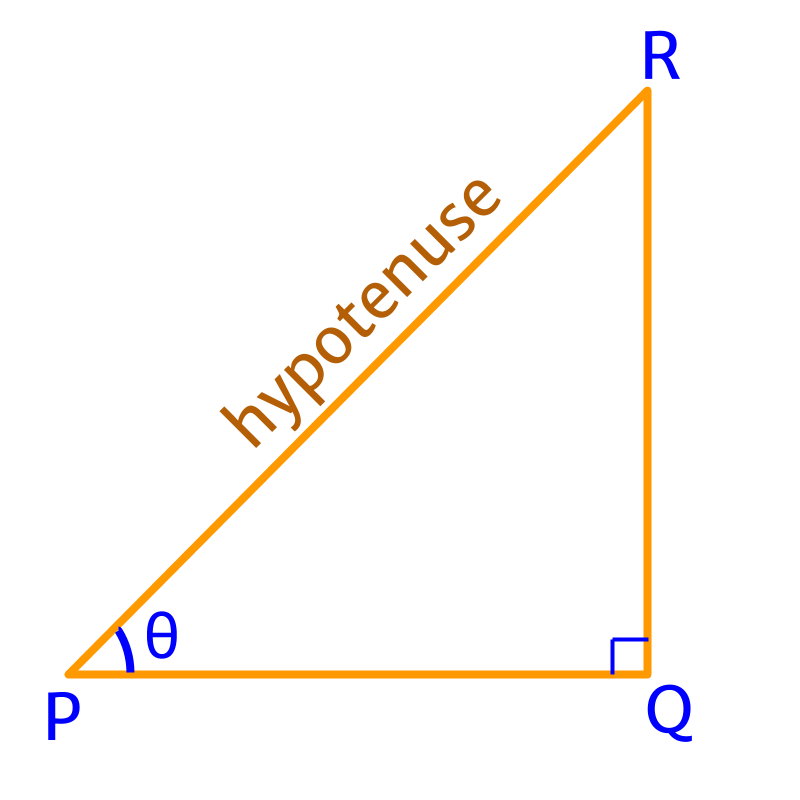In the given figure, $\overline{QR}$$\overline{Q R}$ is the opposite side.

In the given figure, $\overline{PQ}$$\overline{P Q}$ is the adjacent side.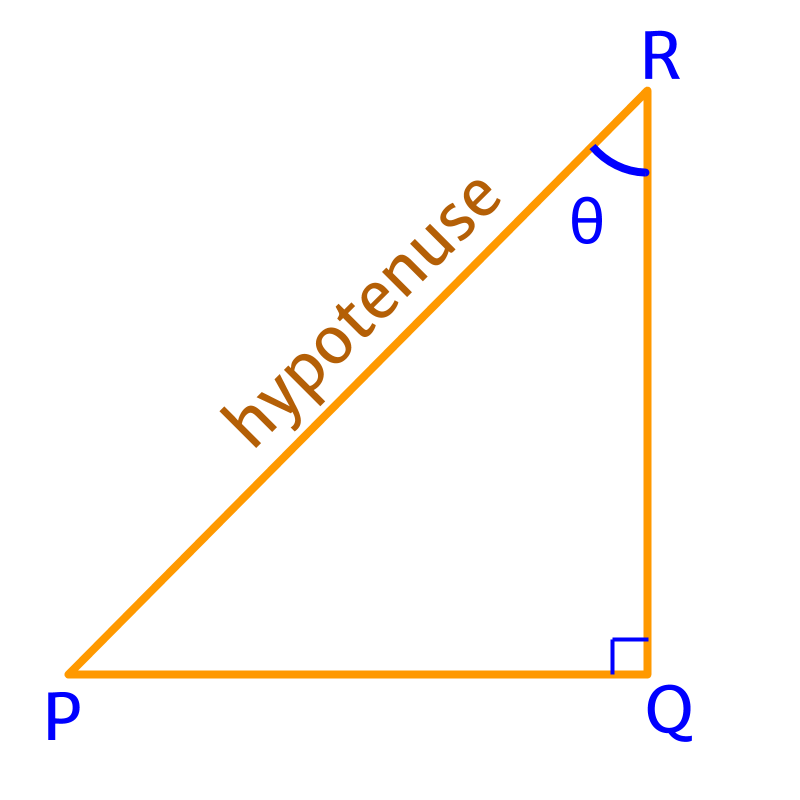In the given figure, the opposite side to $\angle \theta$$\angle \theta$ is $\overline{PQ}$$\overline{P Q}$.

In the given figure, the adjacent side to $\angle \theta$$\angle \theta$ is $\overline{QR}$$\overline{Q R}$.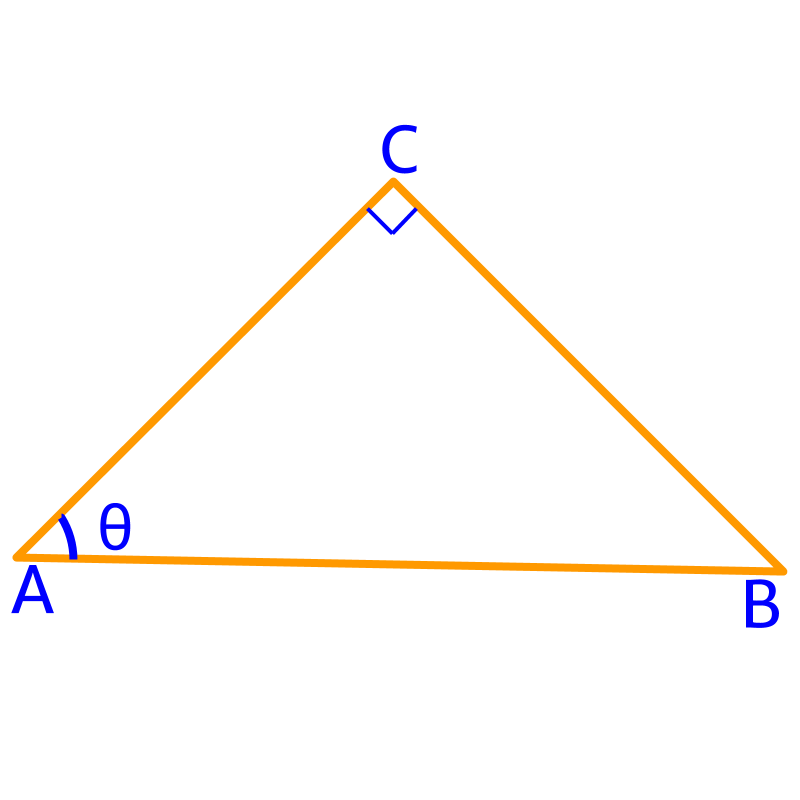In the given figure, the hypotenuse is $\overline{AB}$$\overline{A B}$.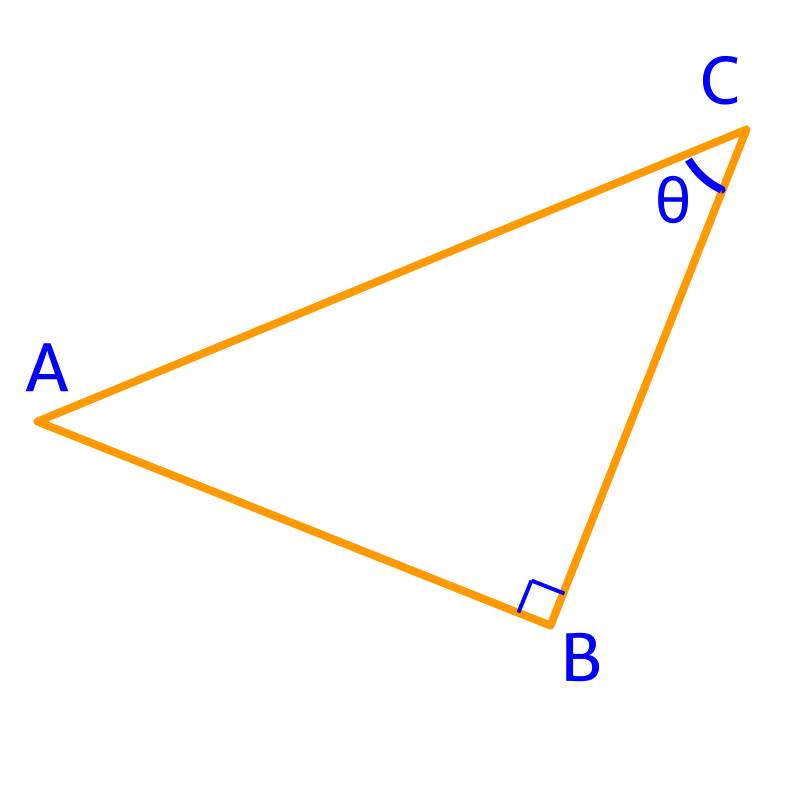In the given figure, the hypotenuse is $\overline{AC}$$\overline{A C}$.In the given figure, the opposite side to $\angle \theta$$\angle \theta$ is $\overline{BC}$$\overline{B C}$.In the given figure, the adjacent side to $\angle \theta$$\angle \theta$ is $\overline{BC}$$\overline{B C}$.

sin, cos, tan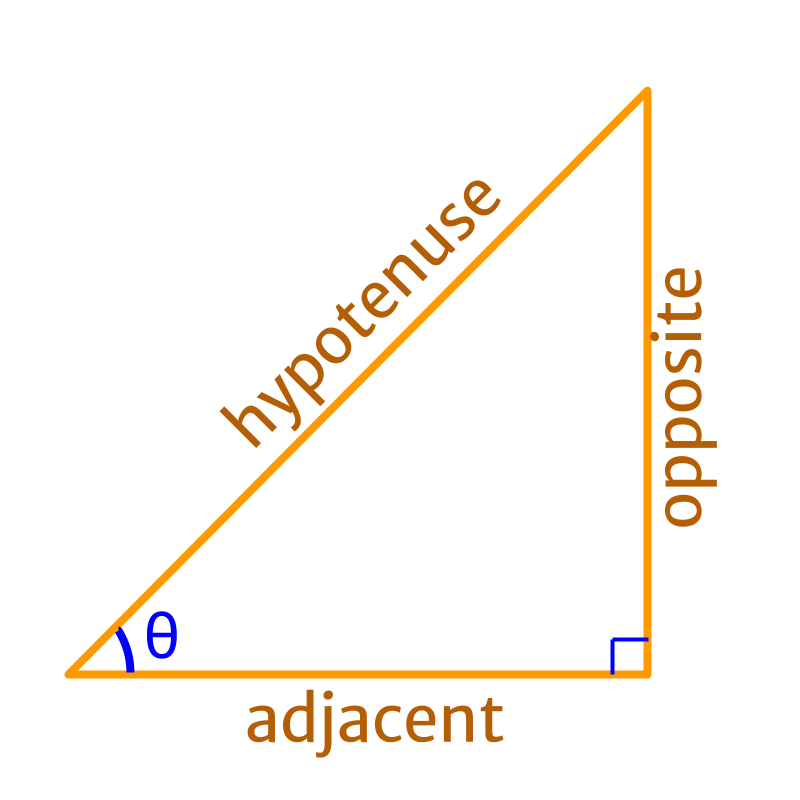$\mathrm{sin}\theta =\frac{\text{opposite}}{\text{hypotenuse}}$$\sin \theta = \frac{\textrm{o p p o s i t e}}{\textrm{h y p o t e \nu s e}}$

$\mathrm{cos}\theta =\frac{\text{adjacent}}{\text{hypotenuse}}$$\cos \theta = \frac{\textrm{a \mathrm{dj} a c e n t}}{\textrm{h y p o t e \nu s e}}$

$\mathrm{tan}\theta =\frac{\text{opposite}}{\text{adjacent}}$$\tan \theta = \frac{\textrm{o p p o s i t e}}{\textrm{a \mathrm{dj} a c e n t}}$

$\mathrm{tan}\theta =\frac{\mathrm{sin}\theta }{\mathrm{cos}\theta }$$\tan \theta = \frac{\sin \theta}{\cos \theta}$

sec, cosec, cot

$\frac{1}{\mathrm{cos}\theta }=\frac{\text{hypotenuse}}{\text{adjacent}}$$\frac{1}{\cos \theta} = \frac{\textrm{h y p o t e \nu s e}}{\textrm{a \mathrm{dj} a c e n t}}$

$\frac{1}{\mathrm{cos}\theta }$$\frac{1}{\cos \theta}$ is called as '$\mathrm{sec}\theta$$\sec \theta$'

$\mathrm{sec}\theta$$\sec \theta$

$\quad \quad = \frac{\textrm{h y p o t e \nu s e}}{\textrm{a \mathrm{dj} a c e n t}}$

$\quad \quad = \frac{1}{\cos \theta}$

$\frac{1}{\mathrm{sin}\theta }=\frac{\text{hypotenuse}}{\text{opposite}}$$\frac{1}{\sin \theta} = \frac{\textrm{h y p o t e \nu s e}}{\textrm{o p p o s i t e}}$

$\frac{1}{\mathrm{sin}\theta }$$\frac{1}{\sin \theta}$ is called as '$\text{cosec}\theta$$\textrm{\cos e c} \theta$' or '$\mathrm{csc}\theta$$\csc \theta$'

$\text{cosec}\theta$$\textrm{\cos e c} \theta$ or $\mathrm{csc}\theta$$\csc \theta$

$\quad \quad = \frac{\textrm{h y p o t e \nu s e}}{\textrm{o p p o s i t e}}$

$\quad \quad = \frac{1}{\sin \theta}$

$\frac{1}{\mathrm{tan}\theta }=\frac{\text{adjacent}}{\text{opposite}}$$\frac{1}{\tan \theta} = \frac{\textrm{a \mathrm{dj} a c e n t}}{\textrm{o p p o s i t e}}$

$\frac{1}{\mathrm{tan}\theta }$$\frac{1}{\tan \theta}$ is called as '$\mathrm{cot}\theta$$\cot \theta$'.

$\text{cot}\theta$$\textrm{\cot} \theta$

$\quad \quad = \frac{\textrm{a \mathrm{dj} a c e n t}}{\textrm{o p p o s i t e}}$

$\quad \quad = \frac{1}{\tan \theta}$

Summary

Trigonometric Ratios:
$\mathrm{sin}\theta =\frac{\text{opposite}}{\text{hypotenuse}}$$\sin \theta = \frac{\textrm{o p p o s i t e}}{\textrm{h y p o t e \nu s e}}$

$\mathrm{cos}\theta =\frac{\text{adjacent}}{\text{hypotenuse}}$$\cos \theta = \frac{\textrm{a \mathrm{dj} a c e n t}}{\textrm{h y p o t e \nu s e}}$

$\mathrm{tan}\theta =\frac{\text{opposite}}{\text{adjacent}}$$\tan \theta = \frac{\textrm{o p p o s i t e}}{\textrm{a \mathrm{dj} a c e n t}}$

$\mathrm{sec}\theta =\frac{\text{hypotenuse}}{\text{adjacent}}$$\sec \theta = \frac{\textrm{h y p o t e \nu s e}}{\textrm{a \mathrm{dj} a c e n t}}$

$\text{cosec}\theta =\frac{\text{hypotenuse}}{\text{opposite}}$$\textrm{\cos e c} \theta = \frac{\textrm{h y p o t e \nu s e}}{\textrm{o p p o s i t e}}$

$\mathrm{cot}\theta =\frac{\text{adjacent}}{\text{opposite}}$$\cot \theta = \frac{\textrm{a \mathrm{dj} a c e n t}}{\textrm{o p p o s i t e}}$

Outline

The outline of material to learn "Basics of Trigonometry" is as follows.

•   Detailed outline of "trigonometry".

→   Basics - Angles

→   Basics - Triangles

→   Importance of Right Angled Triangle

→   Trigonometric Ratio (Basics)

→   Triangular Form of Trigonometric Ratios

→   Introduction to Standard Angles

→   Trigonometric Ratio of Standard Angles

→   Trigonometric Identities

→   Trigonometric Ratios of Complementary Angles